Elektrolyse van water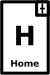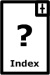Datum: juli 2002

Principe:

 M.b.v. enkele plastic pipetjes bouwen we een Hoffman-elektrolyse apparaat waarmee we de elektrolytische ontleding van water kunnen bestuderen.

Materiaal:

 Plastic pipetjes Alleslijm Water Methylrood (indicator) Kaliumchloride Voedingsbron Multimeter Grafiet (potloodstiften)

Uitvoering:Bouw het Hoffman-electrolyse apparaat op zoals te zien is op de foto hiernaast. Een voorbeeld van een Hoffmann-electrolyse apparaat is te zien in de tekening. Gaten heb ik gesmolten m.b.v. spijkers en schroeven. Na de assemblage heb ik alle verbindingen nagelijmd met 'alleslijm'.  Voor de elektroden gebruiken we grafietstiften uit een vulpotlood. Test voor gebruik of het toestel waterdicht is.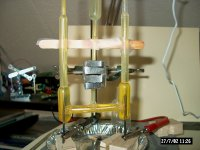Vul het toestel met water (ik heb gewoon leidingwater gebruikt. Voeg enkele korrels methylrood en KCl toe tijden het vullen. Sluit de bovenkanten af (bv. m.b.v. boetseerklei) Verbind de elektroden met een spanningsbron (in mijn geval 13.8 V, 5A).

Meetresultaten en uitwerking:Voor methylrood geldt dat het omslaat bij een pH van 4.2-6.3, de kleur in een zuur milieu is rood en de kleur in een basisch milieu is geel. We zien dat er aan de rechterkant een rode kleur (waar de + pool op was aangesloten) is ontstaan, ten teken dat het milieu daar zuur is. We zien dat er aan de linkerkant (de basische kant, waar de - pool op was aangesloten) meer gas is ontstaan. De verwachte verhouding van 2:1 in termen van gasproductie kunnen we niet echt waarnemen.

Discussie en conclusie:

Elektrolyse is een proces waarbij een chemische reactie wordt uitgevoerd door een elektrische stroom. Bij de elektrolyse van water wordt water geoxideerd aan de anode (negatief) en gereduceerd aan de kathode (positief) volgens de volgende reactie.
 4H2O  +  4e-  -->  4OH-  +  2H2   2H2O  -->  O2  +  4H+  +  4e- kathode anode netto reactie:  6H2O  -->  4OH-  +  4H+  +  2H2  +  O2 hetgeen vereenvoudigd kan worden tot:  2H2O  -->  2H2  +  O2

De kathode kan herkend worden aan de gele kleur van de oplossing, door de OH- productie is deze alkalisch. Tevens kunnen we de kathode herkennen aan de grotere productie van gas. Beide elektrodes produceren gas. De kathode echter produceert twee moleculen waterstof t.o.v. een molecuul zuurstof aan de anode.
Dientengevolge kan de anode herkend worden aan de rode kleur door de productie van H+ hetgeen de oplossing zuur maakt.

Opmerkingen:

 Om een betere geleidbaarheid te verkrijgen heb ik enkele korrels KCl toegevoegd. Het is ook mogelijk om een 9V's batterij als spanningbron te gebruiken. Voor het lijmen kan men het beste een polystyreen lijm gebruiken.

Literatuur:

 Microscale chemistry; John Skinner; Royal Society of Chemistry; 1997; ISBN 1870343492; blz 12-14, 162. Ruud Baars; 'Batterij-scheikunde'; DJO; 1979 10; blz. 309. M.M. Huisman; 'zelf doen: Waaruit bestaat water?'; DJO; 1973 5; blz. 8,9. Relevante websites:

Informatief:

Electrolysis of Water

A standard apparatus for the electrolysis of water is shown in the figure below.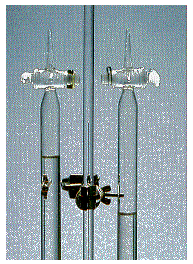electrolysis 2 H2O(l)2 H2(g) + O2(g)

A pair of inert electrodes are sealed in opposite ends of a container designed to collect the H2 and O2 gas given off in this reaction. The electrodes are then connected to a battery or another source of electric current.

By itself, water is a very poor conductor of electricity. We therefore add an electrolyte to water to provide ions that can flow through the solution, thereby completing the electric circuit. The electrolyte must be soluble in water. It should also be relatively inexpensive. Most importantly, it must contain ions that are harder to oxidize or reduce than water.

 2 H2O + 2 e-H2 + 2 OH- Eored = -0.83 V 2 H2OO2 + 4 H+ + 4 e- Eoox = -1.23 V

The following cations are harder to reduce than water: Li+, Rb+, K+, Cs+, Ba2+, Sr2+, Ca2+, Na+, and Mg2+. Two of these cations are more likely candidates than the others because they form inexpensive, soluble salts: Na+ and K+.

The SO42- ion might be the best anion to use because it is the most difficult anion to oxidize. The potential for oxidation of this ion to the peroxydisulfate ion is -2.05 volts.

 2 SO42-S2O82- + 2 e- Eoox = -2.05 V

When an aqueous solution of either Na2SO4 or K2SO4 is electrolyzed in the apparatus shown in the above figure, H2 gas collects at one electrode and O2 gas collects at the other.

What would happen if we added an indicator such as bromothymol blue to this apparatus? Bromothymol blue turns yellow in acidic solutions (pH < 6) and blue in basic solutions (pH > 7.6). According to the equations for the two half-reactions, the indicator should turn yellow at the anode and blue at the cathode.

 Cathode (-): 2 H2O + 2 e-H2 + 2 OH- Anode (+): 2 H2OO2 + 4 H+ + 4 e-

Faraday's law of electrolysis can be stated as follows. The amount of a substance consumed or produced at one of the electrodes in an electrolytic cell is directly proportional to the amount of electricity that passes through the cell.

In order to use Faraday's law we need to recognize the relationship between current, time, and the amount of electric charge that flows through a circuit. By definition, one coulomb of charge is transferred when a 1-amp current flows for 1 second.

1 C = 1 amp-s

Example: To illustrate how Faraday's law can be used, let's calculate the number of grams of sodium metal that will form at the cathode when a 10.0-amp current is passed through molten sodium chloride for a period of 4.00 hours.

We start by calculating the amount of electric charge that flows through the cell.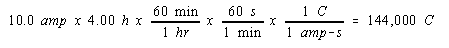Before we can use this information, we need a bridge between this macroscopic quantity and the phenomenon that occurs on the atomic scale. This bridge is represented by Faraday's constant, which describes the number of coulombs of charge carried by a mole of electrons.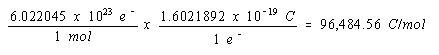Thus, the number of moles of electrons transferred when 144,000 coulombs of electric charge flow through the cell can be calculated as follows.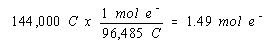According to the balanced equation for the reaction that occurs at the cathode of this cell, we get one mole of sodium for every mole of electrons.

 Cathode (-): Na+ + e-Na

Thus, we get 1.49 moles, or 34.3 grams, of sodium in 4.00 hours.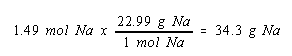The consequences of this calculation are interesting. We would have to run this electrolysis for more than two days to prepare a pound of sodium.

16-01-2017